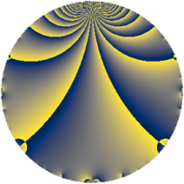# Properties

 Label 31.1.bLevel $31$ Weight $1$ Character orbit 31.b Rep. character $\chi_{31}(30,\cdot)$ Character field $\Q$ Dimension $1$ Newform subspaces $1$ Sturm bound $2$ Trace bound $0$

# Related objects

## Defining parameters

 Level: $$N$$ $$=$$ $$31$$ Weight: $$k$$ $$=$$ $$1$$ Character orbit: $$[\chi]$$ $$=$$ 31.b (of order $$2$$ and degree $$1$$) Character conductor: $$\operatorname{cond}(\chi)$$ $$=$$ $$31$$ Character field: $$\Q$$ Newform subspaces: $$1$$ Sturm bound: $$2$$ Trace bound: $$0$$

## Dimensions

The following table gives the dimensions of various subspaces of $$M_{1}(31, [\chi])$$.

Total New Old
Modular forms 2 2 0
Cusp forms 1 1 0
Eisenstein series 1 1 0

The following table gives the dimensions of subspaces with specified projective image type.

$$D_n$$ $$A_4$$ $$S_4$$ $$A_5$$
Dimension 1 0 0 0

## Trace form

 $$q - q^{2} - q^{5} - q^{7} + q^{8} + q^{9} + O(q^{10})$$ $$q - q^{2} - q^{5} - q^{7} + q^{8} + q^{9} + q^{10} + q^{14} - q^{16} - q^{18} - q^{19} + q^{31} + q^{35} + q^{38} - q^{40} - q^{41} - q^{45} + 2 q^{47} - q^{56} - q^{59} - q^{62} - q^{63} + q^{64} + 2 q^{67} - q^{70} - q^{71} + q^{72} + q^{80} + q^{81} + q^{82} + q^{90} - 2 q^{94} + q^{95} - q^{97} + O(q^{100})$$

## Decomposition of $$S_{1}^{\mathrm{new}}(31, [\chi])$$ into newform subspaces

Label Dim $A$ Field Image CM RM Traces $q$-expansion
$a_{2}$ $a_{3}$ $a_{5}$ $a_{7}$
31.1.b.a $1$ $0.015$ $$\Q$$ $D_{3}$ $$\Q(\sqrt{-31})$$ None $$-1$$ $$0$$ $$-1$$ $$-1$$ $$q-q^{2}-q^{5}-q^{7}+q^{8}+q^{9}+q^{10}+\cdots$$